Author Message
Aslan
Valued Contributor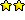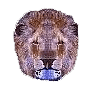Joined: 31 May 2001
Posts: 589
Location: Memphis, TN USAPosted: Mon Apr 02, 2007 11:30 pm    Post subject: How to read from a structure?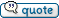How would you read back the following C structure?

Shouldn't the following work or did I do something wrong because all I get in return is a "0" for each variable.

 Code: %%mask = 1    %%iItem = 2    %%state = 3    %%stateMask = 4    %%pszText = 5    %%cchTextMax = 6    %%iImage = 7    %%Lparam = 8    %%iIndent = 9    %%iGroupId = 10   %%LVITEM = @binary(DWORD,%%mask)   %%LVITEM = %%LVITEM@binary(DWORD,%%iItem)   %%LVITEM = %%LVITEM@binary(DWORD,%%ISubItem)   %%LVITEM = %%LVITEM@binary(DWORD,%%state)   %%LVITEM = %%LVITEM@binary(DWORD,%%stateMask)   %%LVITEM = %%LVITEM@binary(DWORD,%%pszText)   %%LVITEM = %%LVITEM@binary(DWORD,%%cchTextMax)   %%LVITEM = %%LVITEM@binary(DWORD,%%iImage)   %%LVITEM = %%LVITEM@binary(DWORD,%%Lparam)   %%LVITEM = %%LVITEM@binary(DWORD,%%iIndent)   %%LVITEM = %%LVITEM@binary(DWORD,%%iGroupId)    %%mask =    %%iItem =    %%state =    %%stateMask =    %%pszText =    %%cchTextMax =    %%iImage =    %%Lparam =    %%iIndent =    %%iGroupId =   %%mask = @val(@BINARY(DWORD,@substr(%%LVITEM,1,4)))   %%iItem = @val(@BINARY(DWORD,@substr(%%LVITEM,5,8)))   %%state = @val(@BINARY(DWORD,@substr(%%LVITEM,9,12)))   %%stateMask = @val(@BINARY(DWORD,@substr(%%LVITEM,13,16)))   %%pszText = @val(@BINARY(DWORD,@substr(%%LVITEM,17,20)))   %%cchTextMax = @val(@BINARY(DWORD,@substr(%%LVITEM,21,24)))   %%iImage = @val(@BINARY(DWORD,@substr(%%LVITEM,25,28)))   %%Lparam = @val(@BINARY(DWORD,@substr(%%LVITEM,29,32)))   %%iIndent = @val(@BINARY(DWORD,@substr(%%LVITEM,33,36)))   %%iGroupId = @val(@BINARY(DWORD,@substr(%%LVITEM,37,40)))     Info %%mask@cr()%%iItem@cr()%%state@cr()%%stateMask@cr()%%pszText@cr()%%cchTextMax@cr()%%iImage@cr()%%Lparam@cr()%%iIndent@cr()%%iGroupId

Aslan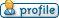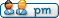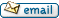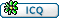Hooligan
VDS Developer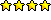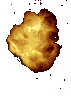Joined: 28 Oct 2003
Posts: 479
Location: CaliforniaPosted: Tue Apr 03, 2007 2:39 am    Post subject:Hi Aslan,

Is this what you are after?

 Code: %%mask = 1    %%iItem = 2    %%state = 3    %%stateMask = 4    %%pszText = 5    %%cchTextMax = 6    %%iImage = 7    %%Lparam = 8    %%iIndent = 9    %%iGroupId = 10   %%LVITEM = @binary(DWORD,%%mask)   %%LVITEM = %%LVITEM@binary(DWORD,%%iItem) #  %%LVITEM = %%LVITEM@binary(DWORD,%%ISubItem)   %%LVITEM = %%LVITEM@binary(DWORD,%%state)   %%LVITEM = %%LVITEM@binary(DWORD,%%stateMask)   %%LVITEM = %%LVITEM@binary(DWORD,%%pszText)   %%LVITEM = %%LVITEM@binary(DWORD,%%cchTextMax)   %%LVITEM = %%LVITEM@binary(DWORD,%%iImage)   %%LVITEM = %%LVITEM@binary(DWORD,%%Lparam)   %%LVITEM = %%LVITEM@binary(DWORD,%%iIndent)   %%LVITEM = %%LVITEM@binary(DWORD,%%iGroupId)      %%mask =    %%iItem =    %%state =    %%stateMask =    %%pszText =    %%cchTextMax =    %%iImage =    %%Lparam =    %%iIndent =    %%iGroupId =   %%mask = @val(@substr(%%LVITEM,1,4))   %%iItem = @val(@substr(%%LVITEM,5,8))   %%state = @val(@substr(%%LVITEM,9,12))   %%stateMask = @val(@substr(%%LVITEM,13,16))   %%pszText = @val(@substr(%%LVITEM,17,20))   %%cchTextMax = @val(@substr(%%LVITEM,21,24))   %%iImage = @val(@substr(%%LVITEM,25,28))   %%Lparam = @val(@substr(%%LVITEM,29,32))   %%iIndent = @val(@substr(%%LVITEM,33,36))   %%iGroupId = @val(@substr(%%LVITEM,37,40))     Info %%mask@cr()%%iItem@cr()%%state@cr()%%stateMask@cr()%%pszText@cr()%%cchTextMax@cr()%%iImage@cr()%%Lparam@cr()%%iIndent@cr()%%iGroupId

_________________
Hooligan

Why be normal?Aslan
Valued ContributorJoined: 31 May 2001
Posts: 589
Location: Memphis, TN USAPosted: Tue Apr 03, 2007 3:59 am    Post subject:Yep, It took several re-reads of the helpfile and some head pounding and I finally ended up with the same code as you.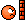hehe.. I see you caught the other error I made tooThanks Hooligan I think I need to walk away from the monitor for a while, it's beginning to blur.vdsalchemist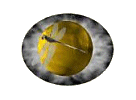Joined: 23 Oct 2001
Posts: 1448
Location: Florida, USAPosted: Tue Apr 03, 2007 1:15 pm    Post subject:Aslan,
Hooligan beat me too it but I can explain why the code below does not work

The @Binary() function take Variable type and a string value. The string value is converted to the specified variable type. When you placed @substr as the parameter it just read the 4 raw bytes from %%LVITEM which the @binary() function could not convert since it was not a plain ASCII string. The @val() function converts the raw bytes back into a string. Since @binary() was returning 0 @val() returned 0.

_________________
Home ofGive VDS a new purpose!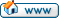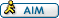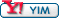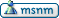Display posts from previous: All Posts1 Day7 Days2 Weeks1 Month3 Months6 Months1 Year Oldest FirstNewest First
 All times are GMT Page 1 of 1